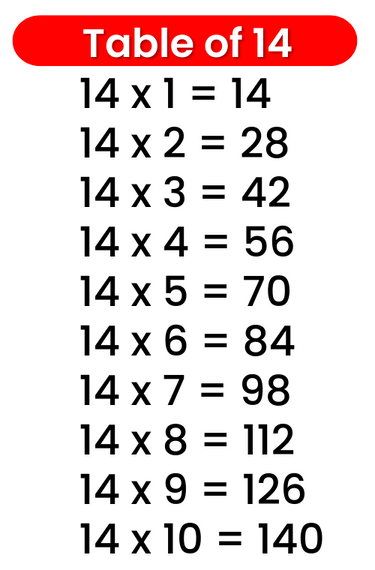# Times Table of 14, 14 ka table

## Times Table of 14, 14 ka table

Maths Multiplication Table of 14, Times Table of 14, 14 ka table, 14 ki Table Chart

14 ×‌ 1 = 14
14 ×‌ 2 = 28
14 ×‌ 3 = 42
14 ×‌ 4 = 56
14 ×‌ 5 = 70
14 ×‌ 6 = 84
14 ×‌ 7 = 98
14 ×‌ 8 = 112
14 ×‌ 9 = 126
14 ×‌ 10 = 140

## 14 ka table### Multiplication Tables of twelve upto 20### Times Table of 14 chart